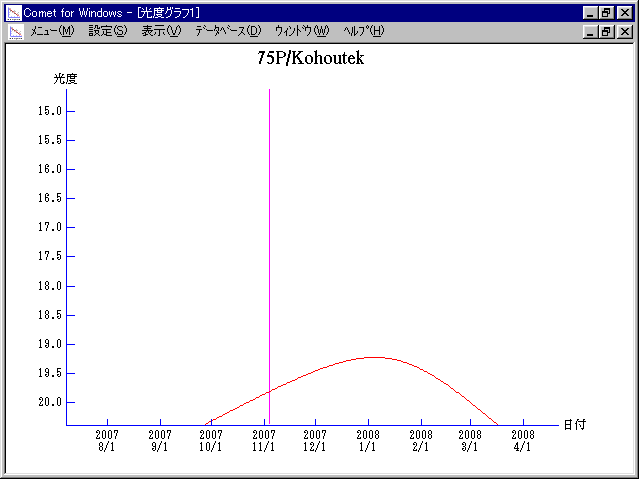# \$B%3%[%&%F%/WB@1(B

75D/Kohoutek (2007)###\$B50F;MWAG(B

```Perturbed ephemeris below is based on elements from MPC 31663.

Epoch = 2454400.50000 TT   T = 2454408.75183 TT
Peri. =  175.62701         a =     3.5528843 AU
Node  =  269.68312 J2000.0 e =     0.4946376
Incl. =    5.90138         q =     1.7954940 AU
```

###\$B8wEYJQ2=(B

```        m1 = 18 + 5 log\$B&\$(B + 5 log r
```##### \$B50F;MWAG\$O(BMinor Planet & Comet Ephemeris Service\$B\$G7W;;\$7\$?\$b\$N\$G\$9!#(B \$B8wEY%0%i%U\$O(BComet for Windows\$B\$G:n@.\$7\$?\$b\$N\$G\$9!#(B